In this lab, we will again make use of the lattice and tidyverse packages:

library(lattice)
library(tidyverse)

## 1. The CDC Data

The Behavioral Risk Factor Surveillance System (BRFSS) is an annual telephone survey of 350,000 people in the United States. As its name implies, the BRFSS is designed to identify risk factors in the adult population and report emerging health trends. For example, respondents are asked about their diet and weekly physical activity, their HIV/AIDS status, possible tobacco use, and even their level of healthcare coverage. The BRFSS Web site (http://www.cdc.gov/brfss) contains a complete description of the survey, including the research questions that motivate the study and many interesting results derived from the data.

We will focus on a random sample of 20,000 people from the BRFSS survey conducted in 2000. While there are over 200 variables in this data set, we will work with a small subset.

The data file can be downloaded at jingshuw.org/materials/stat220/data/cdc.dat. Then set the working directory as in Lab01, and load the data using read.table as follows.

cdc = read.table("cdc.dat", header=TRUE)

If you have trouble setting working directory and loading data file, don’t hesitate to ask TA for help. To view the names of the variables, type the command

str(cdc)

This returns the names genhlth, exerany, hlthplan, smoke100, height, weight, wtdesire, age, and gender. Each one of these variables corresponds to a question that was asked in the survey. For example, for genhlth, respondents were asked to evaluate their general health, responding either excellent, very good, good, fair or poor. The exerany variable indicates whether the respondent exercised in the past month (y) or did not (n). Likewise, hlthplan indicates whether the respondent had some form of health coverage (y) or did not (n). The smoke100 variable indicates whether the respondent had smoked at least 100 cigarettes in her lifetime. The other variables record the respondent’s height in inches, weight in pounds as well as their desired weight, wtdesire, age in years, and gender.

Exercise 1: How many cases are there in this data set? How many variables? For each variable, identify its variable type (e.g. numerical–continuous, numerical– discrete, categorical–ordinal, categorical–nominal).

You could also look at all of the data frame at once by typing its name into the console, but that might be unwise here. We know cdc has 20,000 rows, so viewing the entire data set would mean flooding your screen. It’s better to take small peeks at the data with head, tail or the subsetting techniques that you’ll learn in a moment.

## 2. Review of Lab 2

In Lab 2, we have learned how to find numerical summaries in R, like summary(), mean(), median(), sd() , var() , min() , max() , sum() , IQR(), as well as how to make histograms, boxplots, and scatter plots. As a review, let’s consider a new variable that doesn’t show up directly in this data set: Body Mass Index (BMI)(http://en.wikipedia.org/wiki/Body_mass_index). BMI is a weight to height ratio and can be calculated as:

$\text{BMI} = \frac{\text{weight}~(lb)}{\text{height}~(\text{in})^2} * 703$

703 is the approximate conversion factor to change units from metric (meters and kilograms) to imperial (inches and pounds).

The following two lines first make a new object called bmi and then creates box plots of these values, defining groups by the variable genhlth.

cdc = transform(cdc, bmi = (weight / height^2) * 703)
bwplot(genhlth ~ bmi, data=cdc)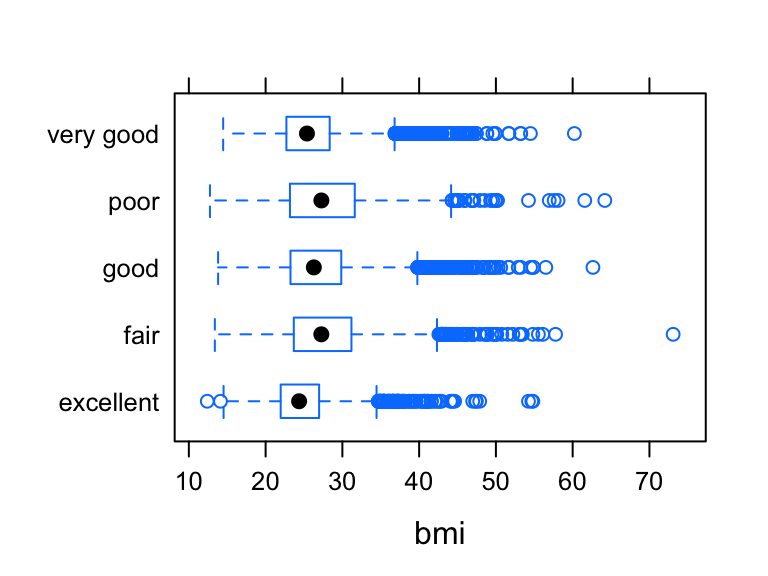Notice genhlth is an ordinal categorical variable that the five levels are ordered: “excellent”, “very good”, “good”, “fair”, and “poor”. We need to tell R that genhlth is ordered, otherwise R is going to order the five levels alphabetically, just as in the boxplot above. We can specify the order of levels using the ordered

cdc = transform(cdc,
genhlth = ordered(genhlth,
levels=c("excellent", "very good", "good", "fair", "poor")))
bwplot(genhlth ~ bmi, data=cdc)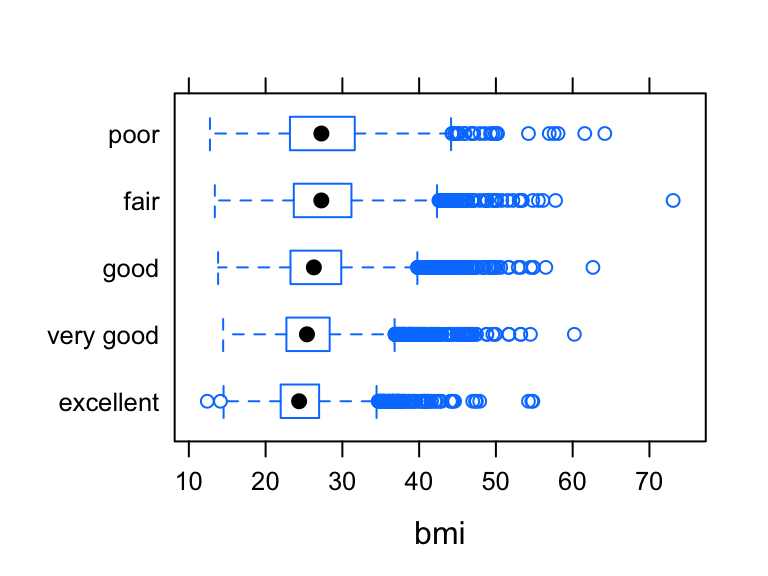Likewise, we can make side-by-side histograms comparing the bmi of people with different self-rated health status.

histogram(~ bmi | genhlth, data=cdc, nint=50, layout = c(1,5))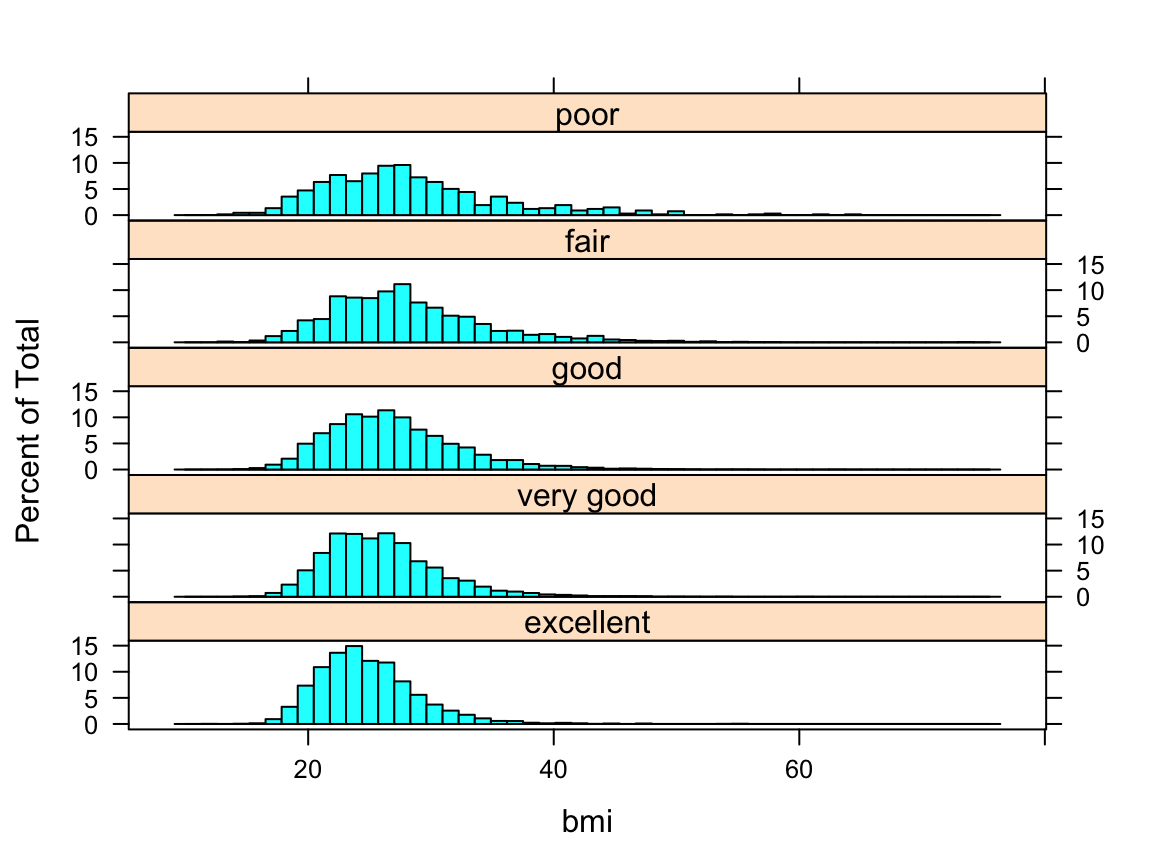We see all 5 histograms are right skewed, and the center of the histograms increase slightly as the health status gets worse.

The following scatterplot of weight versus desired weight.

qplot(weight, wtdesire, data=cdc)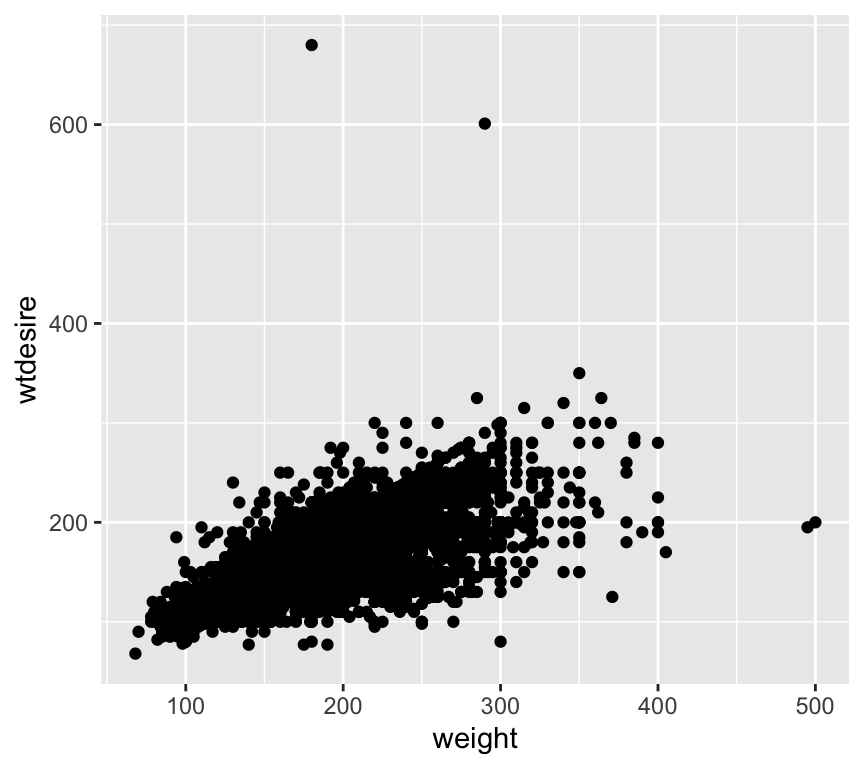Apparently there are two outliers, with incredibly large desired weights (the two guys must be kidding). To remove the two outliers so that we take a closer look at big chunk of points, we can remove these two observation from the plot using subset.

qplot(weight, wtdesire, data=subset(cdc,wtdesire <400), size= I(0.8))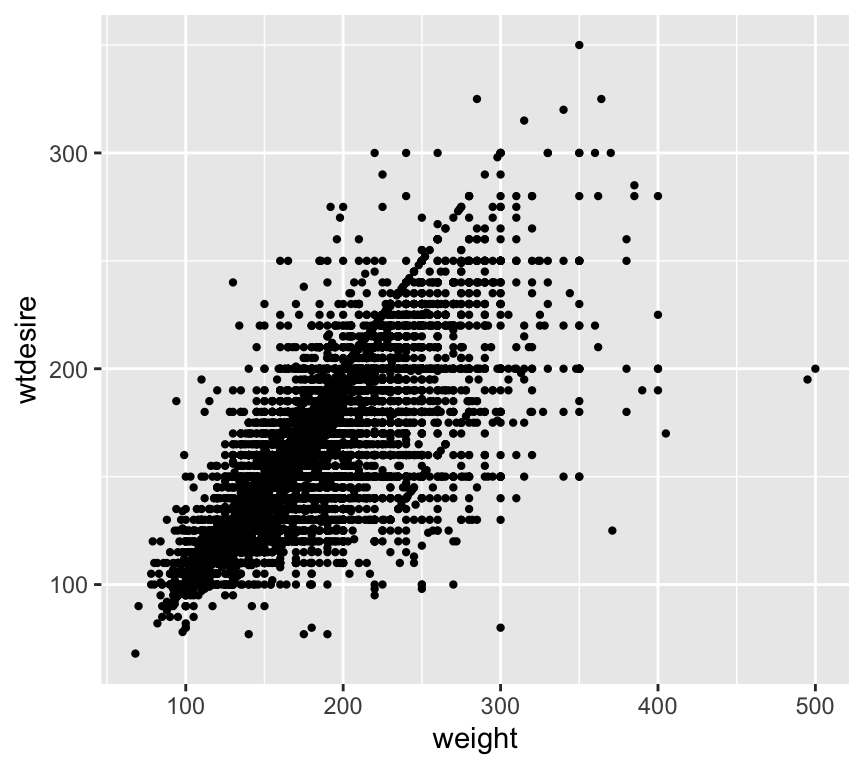Exercise 2: Based on the scatterplot, describe the relationship between these two variables.

One can use color of the point to indicate the gender (or other categorical variables) of the subjects.

qplot(weight, wtdesire, color=gender, data=subset(cdc,wtdesire <400))